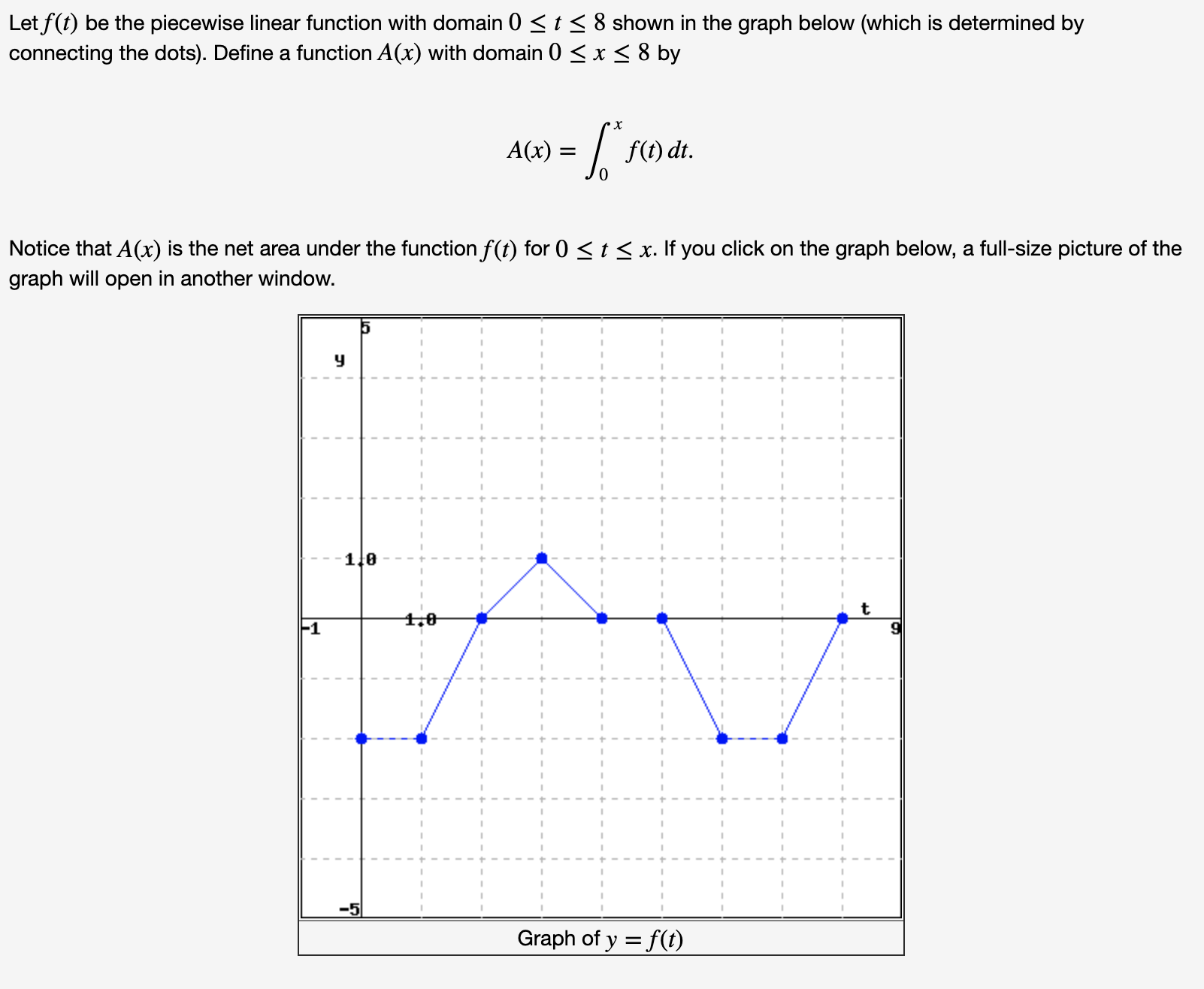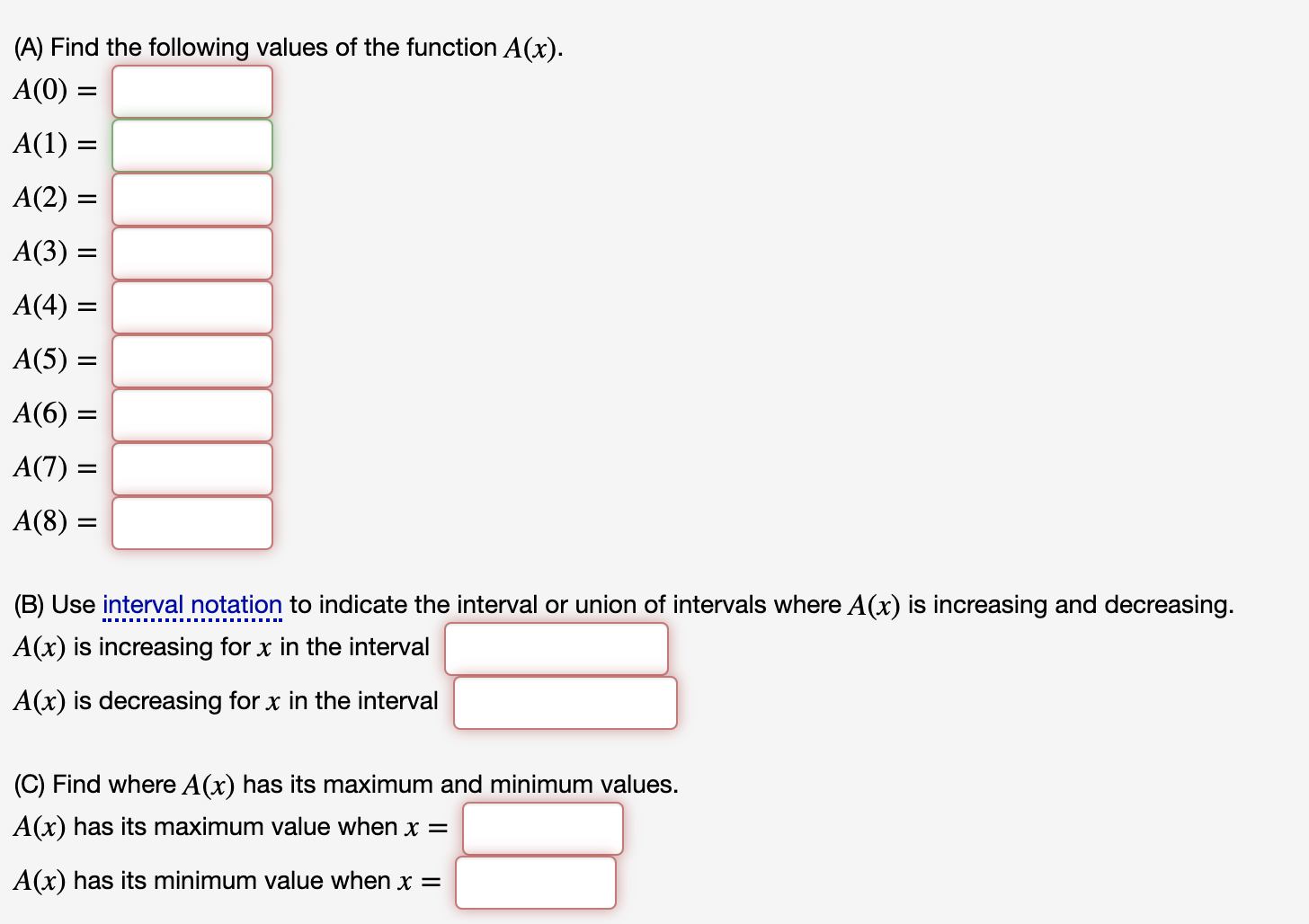Question
32 viewshelp_outlineImage TranscriptioncloseLet f(t) be the piecewise linear function with domain 0help_outlineImage Transcriptionclose(A) Find the following values of the function A(x). A(0) = A(1) = A(2) = A(3) = A(4) = A(5) = A(6) = A(7) = A(8) = (B) Use interval notation to indicate the interval or union of intervals where A(x) is increasing and decreasing. A(x) is increasing for x in the interval A(x) is decreasing for x in the interval (C) Find where A(x) has its maximum and minimum values. A(x) has its maximum value when x = A(x) has its minimum value when x = fullscreen
check_circle

Step 1

By observing the graph, Write the values of the function A(x) at respective x.

Step 2

By observing the graph, Write the intervals at which the function A(x) is increasing.

A(x) is increasing for x in the following intervals:

Step 3

By observing the graph, write the intervals at which the function A(x) is decreasing.

&nbs...

### Want to see the full answer?

See Solution

#### Want to see this answer and more?

Solutions are written by subject experts who are available 24/7. Questions are typically answered within 1 hour.*

See Solution
*Response times may vary by subject and question.
Tagged in

### Calculus